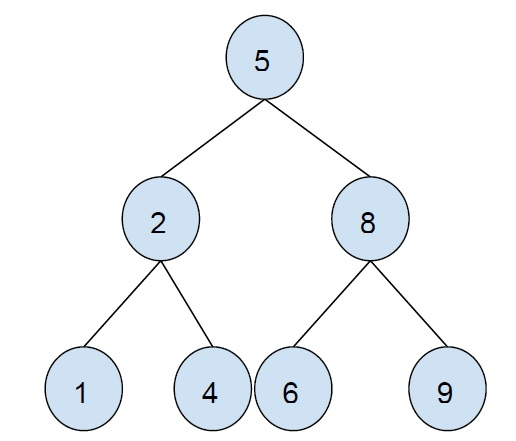# Print all even nodes of Binary Search Tree in C++

In this problem, we are given a binary search tree. Our task is to print all even valued nodes of the binary search tree.

The binary search tree is a binary tree that follows the following condition −

• The left sub-tree always contains nodes with smaller values than the parent node.

• Right, sub-tree always contains nodes with greater values than the parent node.

• All nodes have to follow the above 2 rules.

Example of a binary search tree −Let’s take an example to understand the problem −Output − 2 4 6 8

To solve this problem, we will have to traverse all nodes of the binary search tree and check for the current node’s value. If it is even then print the node otherwise leave it.

## Example

The below code will illustrate the working of our logic −

Live Demo

#include <iostream>
using namespace std;
struct Node {
int key;
struct Node *left, *right;
};
Node* newNode(int item){
Node* temp = new Node;
temp->key = item;
temp->left = temp->right = NULL;
return temp;
}
Node* insertNode(Node* node, int key){
if (node == NULL)
return newNode(key);
if (key < node->key)
node->left = insertNode(node->left, key);
else
node->right = insertNode(node->right, key);
return node;
}
void printEvenNode(Node* root){
if (root != NULL) {
printEvenNode(root->left);
if (root->key % 2 == 0)
cout<<root->key<<"\t";
printEvenNode(root->right);
}
}
int main(){
Node* root = NULL;
root = insertNode(root, 54);
root = insertNode(root, 43);
root = insertNode(root, 12);
root = insertNode(root, 30);
root = insertNode(root, 89);
root = insertNode(root, 67);
root = insertNode(root, 80);
cout<<"All even nodes of the tree are :\n";
printEvenNode(root);
return 0;
}

## Output

All even nodes of the tree are −

12 30 54 80